# Industry News Display

2016-07-26   Control Engineering

## PID loop tuning may not be a hard science, but it’s not magic either. Here are some tuning tips that work.

By: Vance VanDoren, PhD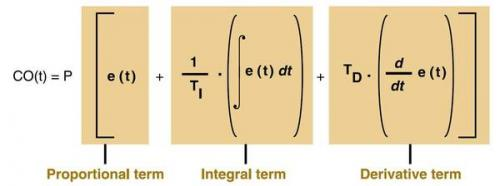A "control loop" is a feedback mechanism that attempts to correct discrepancies between a measured process variable and the desired setpoint. A special-purpose computer known as the "controller" applies the necessary corrective efforts via an actuator that can drive the process variable up or down. A home furnace uses a basic feedback controller to turn the heat up or down if the temperature measured by the thermostat is too low or too high.

For industrial applications, a proportional-integral-derivative (PID) controller tracks the error between the process variable and the setpoint, the integral of recent errors, and the derivative of the error signal. It computes its next corrective effort from a weighted sum of those three terms, then applies the results to the process, and awaits the next measurement. It repeats this measure-decide-actuate loop until the error is eliminated. [subhead]

PID basics

A PID controller using the ideal or International Society of Automation (ISA) standard form of the PID algorithm computes its output CO(t) according to the formula shown in Figure 1. PV(t) is the process variable measured at time t, and the error e(t) is the difference between the process variable and the setpoint. The PID formula weights the proportional term by a factor of P, the integral term by a factor of P/TI, and the derivative term by a factor of P.TD where P is the controller gain, TI is the integral time, and TD is the derivative time.

That terminology bears some explaining. "Gain" refers to the percentage by which the error signal will gain or lose strength as it passes through the controller en route to becoming part of the controller's output. A PID controller with a high gain will tend to generate particularly aggressive corrective efforts.

The "integral time" refers to a hypothetical sequence of events where the error starts at zero, then abruptly jumps to a fixed value. Such an error would cause an instantaneous response from the controller's proportional term and a response from the integral term that starts at zero and increases steadily. The time required for the integral term to catch up to the unchanging proportional term is the integral time TI. A PID controller with a long integral time is more heavily weighted toward proportional action than integral action.

Similarly, the "derivative time" TD is a measure of the relative influence of the derivative term in the PID formula. If the error were to start at zero and begin increasing at a fixed rate, the proportional term would start at zero, while the derivative term assumes a fixed value. The proportional term would then increase steadily until it catches up with the derivative term at the end of the derivative time. A PID controller with a long derivative time is more heavily weighted toward derivative action than proportional action.

Historical note

The first feedback controllers included just the proportional term. For mathematical reasons that only became apparent later on, a P-only controller tends to drive the error downward to a small, but non-zero, value and then quit. Operators observing this phenomenon would manually increase the controller's output until the last vestiges of the error were eliminated. They called this operation "resetting" the controller.

When the integral term was introduced, operators observed that it would tend to perform the reset operation automatically. That is, the controller would augment its proportional action just enough to eliminate the error entirely. Hence, integral action was originally called "automatic reset" and remains labeled that way on some PID controllers to this day. The derivative term was invented shortly thereafter and was described, accurately enough, as "rate control."

Loop tuning is the art of selecting values for the tuning parameters P, TI, and TD so that the controller will be able to eliminate an error quickly without causing the process variable to fluctuate excessively. That's easier said than done.

Consider a car's cruise controller, for example. It can accelerate the car to a desired cruising speed, but not instantaneously. The car's inertia causes a delay between the time that the controller engages the accelerator and the time that the car's speed reaches the setpoint. How well a PID controller performs depends in large part on such lags.

Suppose an overloaded car with an undersized engine suddenly starts up a steep hill. The ensuing error between the car's actual and desired speeds would cause the controller's derivative and proportional actions to kick in immediately. The controller would begin to accelerate the car, but only as fast as the lag allows.

After a while, the integral action would also begin to contribute to the controller's output and eventually come to dominate it because the error decreases so slowly when the lag time is long, and a sustained error is what drives the integral action. But exactly when that would happen and how dominant the integral action would become thereafter would depend on the severity of the lag and the relative sizes of the controller's integral and derivative times.

This simple example demonstrates a fundamental principle of PID tuning. The best choice for each of the tuning parameters P, TI, and TD depends on the values of the other two as well as the behavior of the controlled process. Furthermore, modifying the tuning of any one term affects the performance of the others because the modified controller affects the process, and the process in turn affects the controller.

Ziegler-Nichols tuning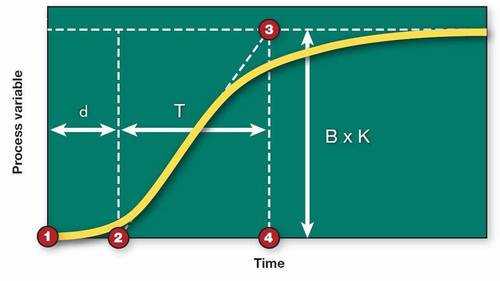How can a control engineer designing a PID loop determine the values for P, TI, and TD that will work best for a particular application? John G. Ziegler and Nathaniel B. Nichols of Taylor Instruments (now part of ABB) addressed that question in 1942 when they published two loop tuning techniques that remain popular to this day.

Their open loop technique is based on the results of a bump or step test for which the controller is taken offline and manually forced to increase its output abruptly. A strip chart of the process variable's subsequent trajectory is known as the "reaction curve" (see Figure 2).

A sloped line drawn tangent to the reaction curve at its steepest point shows how fast the process reacted to the step change in the controller's output. The inverse of this line's slope is the process time constant T, which measures the severity of the lag.

The reaction curve also shows how long it took for the process to demonstrate its initial reaction to the step (the dead time d) and how much the process variable increased relative to the size of the step (the process gain K). By trial-and-error, Ziegler and Nichols determined that the best settings for the tuning parameters P, TI, and TD could be computed from T, d, and K as shown by the equation: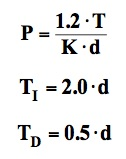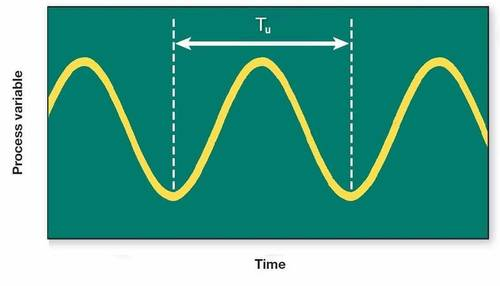After these parameter settings have been loaded into the PID formula and the controller returned to automatic mode, the controller should be able to eliminate future errors without causing the process variable to fluctuate excessively.

Ziegler and Nichols also described a closed loop tuning technique that is conducted with the controller in automatic mode, but with the integral and derivative actions shut off. The controller gain is increased until even the slightest error causes a sustained oscillation in the process variable (see Figure 3).

The smallest controller gain that can cause such an oscillation is called the "ultimate gain" Pu. The period of those oscillations is called the "ultimate period" Tu. The appropriate tuning parameters can be computed from these two values according to the following rules: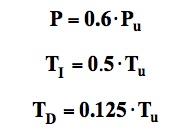Caveats

Unfortunately, PID loop tuning isn't really that simple. Different PID controllers use different versions of the PID formula, and each must be tuned according to the appropriate set of rules. The rules also change when:

• The derivative and/or the integral actions are disabled.
• The process itself is inherently oscillatory.
• The process behaves as if it contains its own integral term (as is the case with level control).
• The dead time d is very small or significantly larger than the time constant T.

Furthermore, Ziegler and Nichols had a particular closed loop performance objective in mind when they settled on their particular tuning rules. They chose to allow some fluctuations in the process variable as long as each successive peak was no more than 0.25 the size of its predecessor, which is called "quarter-wave decay." For applications that require even less fluctuation, additional tweaking of the tuning parameters is required.

That's where loop tuning becomes an art. It takes more than a little experience and sometimes a lot of luck to come up with just the right combination of P, TI, and TD.

Vance VanDoren, PhD, PE, is a Control Engineering contributing content specialist. Reach him at controleng@msn.com. Edited by Jack Smith, content manager, CFE Media, Control Engineering, jsmith@cfemedia.com.

Key concepts

• A PID controller with a high gain tends to generate particularly aggressive corrective efforts.
• Loop tuning is the art of selecting values for tuning parameters that enable the controller to eliminate the error quickly without causing excessive process variable fluctuations.
• Different PID controllers use different versions of the PID formula, and each must be tuned according to the appropriate set of rules.

Consider this

Are PID control loops in your plant optimally tuned?

ONLINE extra